### Combinatorics using Excel formulas

posted Mar 27, 2013, 4:31 PM by Krisztina Szabó   [ updated Dec 10, 2013, 2:18 PM ]

[...] I am not a Frankenstein. I'm a
FrankensTeam
Big thanks to Inga-Kris (the author of this article) because she has collected, translated and explained all these notes.
Special thanks to Olivér Szabó for his help in taking the illustrations playing with his Babylon building toy.

[...] [When called "eee-gore"] No, it's pronounced, "Eye-gore"
The original italian language publications written by Igor-r

## Summary

Using Excel formulas it is possible to build up all the k-element ordered sequences, combinations and permutations of n-element sets for both with or without repetition cases. (Taking into account the limitations of Excel’s calculation methods, in most of the cases n and k should be less than 10.) The article illustrates and explains different formulas from the easiest mechanic copy-down formulas to array-formulas. For all cases I provide downloadable example files.
If you would like to be familiar with the methods of combinatorics and to learn about Excel formulas, start to read the article from the basics. If you need something easier to understand, you can jump to the 2-element cases under Examples section. As a summarization of the cases I developed a dynamic chart to visualize the result sets and the pattern behind the algorithms, so if you would like to see something eye-catching, you can start there.
I developed some ingenious VBA codes too using regular expressions and dictionary objects may be interesting for those who are familiar with RegExp and interested in some less known mechanisms.

## Introduction

Enumerative (basic) combinatorics deals with choosing, grouping and sorting elements of a set according to a rule, so to count and list for example sequences, combinations, permutations and derangements. This article would like to show you how Excel formulas can be used in this field - mainly for listing the possible results.
I started to think about it after reading a discussion on italian NG forum, and soon it became a challenge and passion to build up all the formulas. During the work, I created lot of files, but here I collected the most simple and most beautiful formulas only - however I am sure it is only the top of the iceberg. :-)
I try to present here many different techniques and tricks, and I hope you will find it interesting.
In my explanations and examples I use two constants:
• “n” counts the elements of the base set
• “k” counts the elements we choose from n
In some cases k = n - when we use all the elements of n, for example in case of derangements.
In other cases k could be greater than n if the rule makes possible to choose the same element more times (these are the “with repetition” cases).
Important to note that because of the limitations of the calculation methods (powers of 10), in most of the cases n and k must be less than 10.I would like to start the presentation with the formulas developed to create sequences with repetitions - this is the largest result set (with n^k possibilities), all the others (sequences without repetition, combinations and permutations) are sub-sets of it, so in most of the cases I will use the result of it as a base list and select the appropriate elements according to the rules of the actual task.

Let’s see now how it works!

## The base formulas

### Sequences with repetitions

IT: Disposizioni con ripetizioni
HUN: Ismétléses variáció
ENG: Sequences (ordered lists) with repetitions

When we create sequences with repetition, we choose all the possible k-elements sequences could be formed using the elements of the n-element set. In this “with repetition” case it is possible to use each element of the set several times (maximum k times.)
In this article “sequence” means ordered arrangement, so we take the order of elements into account.Illustration: k=2 element sequences of n=3 element {Red, White, Green}

For example the k=2 element sequences with repetition of the elements of {1,2,3,4,5} are:
11, 12, 13, 14, 15, 21, 22, 23, 24, 25, 31, 32, 33, 34, 35, 41, 42, 43, 44, 45, 51, 52, 53, 54, 55.
The total number of sequences is: =n^k . k could be greater than n because of the possible repetitions.
There are several different ways to list all the sequences using Excel formulas.
First I show you how to list the sequences on a worksheet, then we will change these formulas to produce an array-result.
To make the formulas easier to understand I define two names: k and n according to the mathematical definition:
• “k” is the number of elements of the sequence, (in the below example, it is cell A4)
• “n” is the number of different elements of the set (in the below example, it is cell B4)

#### Simple copy-down formulas

The first formula I propose returns the sequences as decimal numbers in individual cells:

`=MMULT(1+MOD(INT((ROW(A1)-1)/(n^(COLUMN(OFFSET(\$A\$1,,,,k))-1))),n),10^(ROW(OFFSET(\$A\$1,,,k))-1))`
Write in a cell and copy down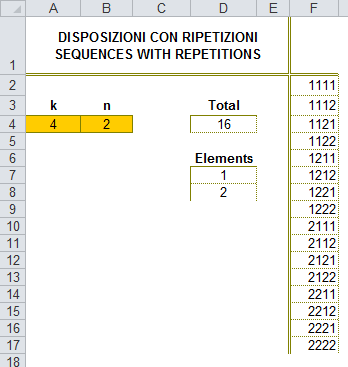Picture 1

It is a "mechanic" formula works using relative cell reference in ROW(A1). If it is dragged over more cells than the total number of sequences (n^k) it will repeat the values ​​again from the first sequence.

[If you want to limit the results you only need to add a condition using an IF function: if the row index exceeds n^k, add empty string or 0 or an error value according to your needs.
Here is an example resulting #NA! instead of repeated sequences:

```=IF(ROW(A1)>n^k,NA(),MMULT(1+MOD(INT((ROW(A1)-1)/(n^(COLUMN(OFFSET(\$A\$1,,,,k))-1))),n), ````10^(ROW(OFFSET(\$A\$1,,,k))-1)))`

Or another solution (I used this in the example file too), leaving the formula as it was originally, you can add conditional formatting to show useful results:
select the entire range of formulas. In the Conditional Formatting menu, use this formula:

`=ROW(A1)>n^k `

and set the font color to white.]

The base formula above is a multiplication of two matrixes. The first matrix is ​​the most important element of the solution, because this part generates the unique sequences. The multiplication is only necessary to “combine” the elements of the sequence to decimal numbers. Accordingly it is possible to use the first matrix as a standalone formula: this way the result is unique numbers in cells (columns) therefore the formula will work for cases n>=10 too. Replace the nested OFFSET formula within COLUMN by a relative column reference (A\$1) and copy it down and right:

`=1+MOD(INT((ROW(\$A1)-1)/(n^(COLUMN(A\$1)-1))),n)`

The result of this formula for n=2 k=4 case: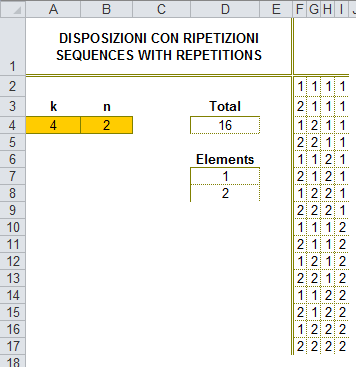Picture 2

[In this case it is also possible to delimit the useful result in various ways, but it will be needed to take into account the number of columns too. In the attached file I use conditional formatting for the entire range containing formulas. In the  Conditional Formatting menu use this formula:

`=OR(COLUMN(A\$1)>k,ROW(\$A2)>n^k)`

and set the font color to white.]

#### Detailed explanation

Let me explain the solutions a little bit more, because I will use this logic many times in the following parts. It will be easy to understand what the formula does if you see the simple pattern of the result - imagine how you would write the sequences manually. In the first column on picture 2 (column F) the numbers are repeated one time (2^0) in the second column they are repeated two times (2^1) then 4 times (2^2) and 8 times (2^3) so we repeat the n numbers according to the powers of n. Mathematically INT and MOD functions could be used to create the result, the simplest way is this copy down and right formula:

`=1+MOD(INT((ROW(\$A1)-1)/(n^(COLUMN(A\$1)-1))),n)`

You can see that k is not used in this formula, because it is only a limit for the powers, that is why I use k in the above mentioned conditional formatting formula - it is a way to hide the unwanted columns (> k).

If you would like to have the sequences as decimal numbers - as it is in the result of the very first formula (picture 1) - you only need to multiply the numbers with the powers of 10 and sum it - using matrix-multiplication (MMULT). To use MMULT the numbers coming from the above mentioned formula must be combined into a one-row matrix, so instead of referencing only A\$1 I use an OFFSET function with k columns:

`=1+MOD(INT((ROW(A1)-1)/(n^(COLUMN( OFFSET(\$A\$1,,,,k))-1))),n)`

For example, the k=4 elements of the sixth row is this matrix: {2, 1, 2, 1}

The second argument of MMULT in the original formula:

`10 ^ (ROW (OFFSET (\$A\$1,, k)) -1) `

returns an array containing the successive powers of 10. So in the case of k = 4, it returns:

`{1 \ 10 \ 100 \ 1000}`

as a one-column matrix. (As a variation, you can choose this version resulting reverse order of powers of 10:

`0.1^(ROW(OFFSET(\$A\$1,,,k))-k) `

resulting:

`{1000\100\10\1}`

MMULT gives us the the final result by multiplying the unique numbers with the appropriate power of 10:

`2 * 1 + 1 * 10 + 2 * 100 + 1 * 1000 = 1212 `

(see sixth line, shown in Picture 1).

Now you can understand how this formula works:

`=MMULT(1+MOD(INT((ROW(A1)-1)/(n^(COLUMN(OFFSET(\$A\$1,,,,k))-1))),n),```` 10^(ROW(OFFSET(\$A\$1,,,k))-1))```

Example file: Sequences_with_repetition_formule.xlsx

#### Formulas producing array result - the base matrixes

Example file 1: Sequences_with_repetition_name_disp_1.xlsx
Example file 2: Sequences_with_repetition_name_disp_2.xlsx

The above explained formulas are mechanic “copy down” formulas using relative cell references thus they are limited to be used in worksheet cells and could not give array result containing all the sequences. To have this kind of result a matrix formula is needed, so I change the copy-down formulas replacing the cell references with correctly dimensioned ranges: n^k rows and k columns. Here I use OFFSET function, but INDEX could also be a solution as you will see in an example later.
I will define these formulas as named formulas because in the coming sections I will use these matrixes as base lists of my further investigations.

[How to define Names (in Excel 2007 or newer): Ctrl+F3 (or: Formulas / Name Manager) click New and type the name to the Name field, type/paste the formula to the Refers to field.]

The first name (disp_1) is based on the first formula producing the sequences as decimal numbers:

Name: disp_1

```=MMULT(1+MOD(INT((ROW(OFFSET(\$A\$1,,,n^k))-1)/(n^(COLUMN(OFFSET(\$A\$1,,,,k))-1))),n), ````10^(ROW(OFFSET(\$A\$1,,,k))-1))`

or the variation with reverse order of powers of 10:

```=MMULT(1+MOD(INT((ROW(OFFSET(\$A\$1,,,n^k))-1)/(n^(COLUMN(OFFSET(\$A\$1,,,,k))-1))),n), ````0.1^(ROW(OFFSET(\$A\$1,,,k))-k))`

The result is a matrix with n^k rows and one column.

Example file: Sequences_with_repetition_name_disp_1.xlsx

The second name (disp_2) is based on the second formula producing the elements of the sequences as separated numbers, so in separated columns:

Name: disp_2

`=1+MOD(INT((ROW(OFFSET(\$A\$1,,,n^k))-1)/(n^(COLUMN(OFFSET(\$A\$1,,,,k))-1))),n)`

The result is a matrix with n^k rows and k columns, containing the elements of the sequences with repetition as separated numbers in its columns.

Example file: Sequences_with_repetition_name_disp_2.xlsx

To see the result on the worksheet, you need to array-enter these names into a range of cells:

For disp_1 first select a range in a column high enough to place the result (in the example file F2 : F1000) type in the formula bar = disp_1 and confirm with Ctrl+Shift+Enter.

For disp_2 select a range large enough in rows and columns (in the example file F2 : N1000) then type in the formula bar = disp_2 and confirm with Ctrl+Shift+Enter.

The results are visibly similar to the images 1 and 2. One difference is that the cells out of the (n^k x k) matrix, return #N/A! error because the result matrix is correctly dimensioned. That is why we can use these matrixes in the following solutions.

### Sequences without repetition

IT: Disposizioni semplici (senza ripetizioni)
HUN: Ismétlés nélküli variáció
EN: Sequences without repetition

A k-element sequence without repetition is an ordered sub-set of an n-element set in which no element occurs more than once.Illustration: k=2 element sequence without repetition of {Red, White, Green}

For example, 2 element sequences of the set formed by 5 elements {1,2,3,4,5} are: 12, 13, 14, 15, 21, 23, 24, 25, 31, 32, 34, 35, 41, 42, 43, 45, 51, 52, 53, 54. The formula to calculate the number of sequences:

`=FACT(n)/FACT(n-k)`

or:
`=PERMUT(n,k)`

In the example the number of sequences for k=2, n=5: 5! / (5-2)! = 5! / 3! = 120/6 = 20
Permutations (without repetition) are special cases of sequences: permutations of an n-element set are the n-element sequences without repetitions. In other words, when creating sequences without repetitions in k=n case, we create the permutations of the n element. The total number of permutations of an n element set is n! (The factorial of 0 is defined to be 1.)
There are some different ways to create formulas resulting the sequences. I will show you formulas using help columns and one standalone formula too, without using any help cells. Both way is based on the sequences with repetition - the challenge is how to leave out those elements which contain duplicated values.

#### Formula using help column

Example file: Sequences_without_repetition_support.xlsx

This array-entered copy-down formula will be the help column testing if there are duplications within the sequences with repetition:

`=IF(ISNA(MODE(MOD(INT((ROW(A1)-1)/(n^(COLUMN(OFFSET(A1,,,,k))-1))),n))),`
`MMULT(1+MOD(INT((ROW(A1)-1)/(n^(COLUMN(OFFSET(\$A\$1,,,,k))-1))),n),`
`10^(ROW(OFFSET(\$A\$1,,,k))-1)))`

Confirm with Control+Shift+Enter and copy down

The idea is very simple: I calculate the digits using the formula you could already be familiar with, and I try to find the mode of the numbers. If mode does not exists or in other words, the data set contains no duplications, Excel’s MODE function gives #NA! error. In this case I calculate the value. I do not use the <value_if_false> part of the IF formula, because this way it results FALSE for the duplicated cases, and in the next column I simply need a SMALL function to sort the result - knowing that SMALL ignores boolean values.

`=SMALL(OFFSET(\$F\$2,,,n^k),ROW(A1))`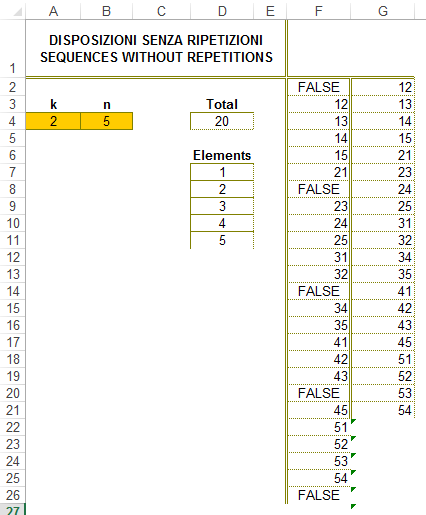Picture 3

#### Formula without help column

This section is based on the article: https://sites.google.com/site/e90e50fx/home/disposizioni-semplici-senza-ripetizioni
Example file: Sequences_without_repetition_no_support.xlsx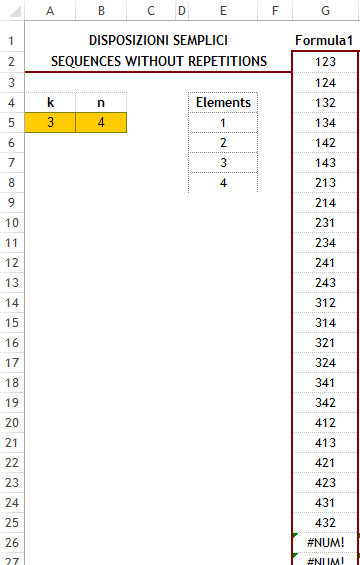Picture 4

It is possible to build a formula producing the sequences without repetition without using any help cells. The logic used in the formula is very simple: I test the values of sequences with repetition with the help of the powers of 10:

`121 -> 10^1 + 10^2 + 10^1 -> 120`
`122 -> 10^1 + 10^2 + 10^2 -> 210`
`123 -> 10^1 + 10^2 + 10^3 -> 1110`
`124 -> 10^1 + 10^2 + 10^4 -> 10110 `

As you can see for the numbers contain no duplicated values, the result of the test contains only 0 or 1 and the number of 1s is equal to the number of digits, which is k in our case. It is enough to substitute the 0s with empty string and test if the result is “1” repeated k times:

`=REPT(1,k)`

Using the idea this is the array formula I developed:

`=SMALL(IF(SUBSTITUTE(MMULT(10^(1+MOD(INT((ROW(OFFSET(\$A\$1,,,n^k))-1)/`
`(n^(COLUMN(OFFSET(\$A\$1,,,,k))-1))),n)),ROW(OFFSET(\$A\$1,,,k))^0),0,"")=REPT(1,k),`
`MMULT(1+MOD(INT((ROW(OFFSET(\$A\$1,,,n^k))-1)/(n^(COLUMN(OFFSET(\$A\$1,,,,k))-1))),n),`
`10^(ROW(OFFSET(\$A\$1,,,k))-1))),ROW(A1))`

Write in a cell, confirm with Ctrl+Shift+Enter and copy down

To make it easier to read, I use the name disp_2 as defined above:

`=1+MOD(INT((ROW(OFFSET(\$A\$1,,,n^k))-1)/(n^(COLUMN(OFFSET(\$A\$1,,,,k))-1))),n)`

(Remember, this is the formula resulting a matrix with n^k rows and k columns, the elements of the sequences with repetition are placed in the columns.) So using this name the formula looks simpler and focuses on the duplication test:

`=SMALL(IF(SUBSTITUTE(MMULT(10^(disp_2),ROW(OFFSET(\$A\$1,,,k))^0),0,"")=REPT(1,k),`
`MMULT(disp_2,10^(ROW(OFFSET(\$A\$1,,,k))-1))),ROW(A1))`

Or a bit more easier using disp_1 too instead of MMULT:

`=SMALL(IF(SUBSTITUTE(MMULT(10^(disp_2),ROW(OFFSET(\$A\$1,,,k))^0),0,"")=REPT(1,k),disp_1),`
`ROW(A1))`

If the test is true, or in other words, the sequence does not contain duplicated values, I matrix-multiply disp_2 with the powers of 10 (disp_1). I did not fill use the <value_if_false> part of the IF function because this way IF results simply FALSE logical value when the condition does not meet. Then I use SMALL to sort this result and have the values at the top, separated from the FALSE logical values.

### Combinations without repetition

IT: Combinazioni semplici (senza ripetizioni)
HUN: Ismétlés nélküli kombináció
EN: Combinations without repetition

A k element combination of a n-element set is a subset of k distinct elements where the order of the elements does not matter (unlike permutations). In other words, if two case are differ only in the order of the elements, then this cases are regarded as same. The number of possible k-combinations is equal to the binomial coefficient:

`n! / (n-k)!*k! where k<=n `

or using the Excel formula:

`=COMBIN(n,k)`

As an example, the number of 4-element combinations of six numbers {1,2,3,4,5,6}  is 6!/(4!2!) = 15

The combinations written in 4-digit number format: 1234, 1235, 1236, 1245, 1246, 1256, 1345, 1346, 1356, 1456, 2345, 2346, 2356, 2456, 3456.Illustration: k=2 element combination without repetition of {Yellow, Green, Red, Blue}

To list all the combinations I show here two solutions. Both based on the list of sequences with repetition, but I found two different methods how to test which values meet the requirement of combinations without repetition.

#### First solution: using frequency test

Example file: Combinations_without_repetition_frequency_test_disp_1.xlsx
Example file: Combinations_without_repetition_frequency_test_disp_2.xlsx

Remember that we have a list of sequences with repetitions of which we would like to eliminate the repeated values and we need only one value consists of the same elements, namely to eliminate the permutations too. In the previous section I used the powers of 10 to leave out the repeated values. Now I will use the same logic for testing if numbers consist of the same digits.
As a simple example take this two arrangements: 1, 2 and 2, 1; both are combination of 1 and 2 and appears as different permutations.
Note that:
`10 ^ 1 +10 ^ 2 = 10 ^ 2 +10 ^ 1 `
so for the sequences 12 and 21 the result of this test is 120. Evidently the permutations of the same elements result the same test value.
If we calculate these values for all combinations, we can realize a simple rule that identifies the combinations we need: those are the combinations for which the test value occures k! (k factorial) times within all the test values - because the number of permutations of k elements is k!.
So we need those combinations where the frequency of the test value is k! and we would like to have only one of these combinations - FREQUENCY formula do exactly what we need: its way of working identifies the unique values, we only need to check if it is = k!
In practice it is not as complicated as it sounds. If you take a look at my files and test parts of the formulas using F9 you will see the logic behind.
After building up the test, the structure of the formula is the same: using an IF to select the appropriate values then sort it with SMALL.

Here is my formula using the name disp_2 defined above:

`=SMALL(IF(FREQUENCY(MMULT(10^disp_2,ROW(OFFSET(\$A\$1,,,k))^0),`
`MMULT(10^disp_2,ROW(OFFSET(\$A\$1,,,k))^0))=FACT(k),`
`MMULT(disp_2,10^(ROW(OFFSET(\$A\$1,,,k))-1))),ROW(A1)) `
Write in a cell and copy down

Example file: Combinations_without_repetition_frequency_test_disp_2.xlsx

Using name disp_1 there is an additional step to separate the digits using MID:

`=SMALL(IF(FREQUENCY(MMULT(10^MID(disp_1,COLUMN(OFFSET(\$A\$1,,,,k)),1),`
`ROW(OFFSET(D1,,,k))^0),MMULT(10^MID(disp_1,COLUMN(OFFSET(\$A\$1,,,,k)),1),`
`ROW(OFFSET(\$A\$1,,,k))^0))=FACT(k),disp_1),ROW(A1)) `
Write in a cell and copy down

Example file: Combinations_without_repetition_frequency_test_disp_1.xlsx

#### Second solution: testing the ascending order

Example file: Combinations_without_repetition_order_test.xlsx

The logic of this solution is easier than the frequency-test in the first solution. The question is the same: we have k! pieces of identical solutions which are permutations of each other - how it is possible to choose one of these to represent a combination? The idea came into my mind while I was reading a definition of combinations on Wikipedia: You can choose between the different permutations of the same set by the order of its elements - choose the one in which the components appear in ascending order.
So essentially if we consider the two sequences: 1, 2, 3 and 2, 1, 3 it is sufficient to choose the one in which the objects are sorted in ascending order: 1, 2, 3. This rule is also a good way to exclude the sequences with repetitions. For example 1; 1; 2 (where 1 duplicated) is not a combination because the inequality is not true: 1 < 1 < 2, while in the arrangement 1, 2, 3, the inequality 1 < 2 < 3 is true.
In Excel the easiest way to test if 1, 2, 3 is in ascending order is:
`{1, 2} <{2, 3} = {TRUE, TRUE} `

so comparing the first k-1 elements with the last k-1.
It will be easy to adapt in our formulas. The above mentioned named formula disp_2 creates the matrix of the sequences. With a small modification I make it produce the first and last k-1 elements.
`=1+MOD(INT((ROW(OFFSET(\$A\$1,,,n^k))-1)/(n^(COLUMN(OFFSET(\$A\$1,,,,k))-1))),n) `
The highlighted OFFSET part can easily be modified to give back the last k-1 elements, simply shift the reference one column right and minus 1 from the k parameter:
`OFFSET(\$A\$1,,,,k-1)`` - for the first k-1 elements `
`OFFSET(\$B\$1,,,,k-1)`` - for the last k-1 elements `
In the final formula I compare (<) the two matrixes. (I left out the 1+ part from the beginning - it is unnecessary in the comparison.) I will use the value from the well-known array of sequences with repetition if the condition is true (the “sum” (MMULT) of the TRUE values = k-1), then I use the SMALL function to sort the result.
Here is the final formula - could also be learned in the example file: Combinations_without_repetition_order_test.xlsx

`=SMALL(IF((MMULT(--(MOD(INT((ROW(OFFSET(\$A\$1,,,n^k))-1)/`
`(n^(COLUMN(OFFSET(\$A\$1,,,,k-1))-1))),n) < MOD(INT((ROW(OFFSET(\$A\$1,,,n^k))-1)/`
`(n^(COLUMN(OFFSET(\$B\$1,,,,k-1))-1))),n)),ROW(OFFSET(\$A\$1,,,k-1))^0)=(k-1)),`
`MMULT(1+MOD(INT((ROW(OFFSET(\$A\$1,,,n^k))-1)/(n^(COLUMN(OFFSET(\$A\$1,,,,k))-1))),n),`
`0.1^(ROW(OFFSET(\$A\$1,,,k))-k))),ROW(A1)) `
Write in a cell, confirm with Ctrl+Shift+Enter and copy down

The highlighted part of the formula could be replaced with the original disp_2 name.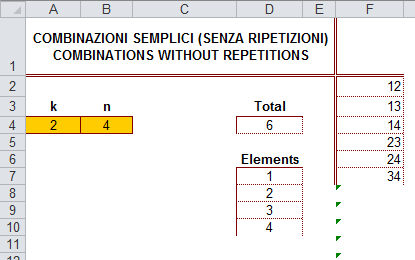Picture 5

These illustrative explanations could help to understand the formulas. In the example files using the formula evaluate tool and F9 you can dig into more details if you need a deeper understanding.

### Combinations with repetitions

IT: Combinazioni con ripetizione
HUN: Ismétléses kombináció
EN: Combinations with repetitions

If we allow duplications in the combinations, so it is possible to choose one element more than once from the n-element set, but order of the elements does not matter, we talk about combinations with repetitions.
Formula to calculate the total number of this kind of combinations:
`=FACT(n+k-1) / ( FACT(n-1) * FACT(k) ) `Illustration: k=2 element combination with repetition of {Yellow, Green, Red, Blue}

Fortunately only a slight change is needed in the formula of simple combinations to accommodate it to repetitions. It should choose all sequences in which the elements are ordered, so that each element is less than or equal to the following element. In the formula only need to replace “<” with “<=”:

`=SMALL(IF((MMULT(--(MOD(INT((ROW(OFFSET(\$A\$1,,,n^k))-1)/`
`(n^(COLUMN(OFFSET(\$A\$1,,,,k-1))-1))),n)<=MOD(INT((ROW(OFFSET(\$A\$1,,,n^k))-1)/`
`(n^(COLUMN(OFFSET(\$B\$1,,,,k-1))-1))),n)),ROW(OFFSET(\$A\$1,,,k-1))^0)=(k-1)),`
`MMULT(1+MOD(INT((ROW(OFFSET(\$A\$1,,,n^k))-1)/(n^(COLUMN(OFFSET(\$A\$1,,,,k))-1))),n),`
`0.1^(ROW(OFFSET(\$A\$1,,,k))-k))),ROW(A1)) `
Write in a cell, confirm with Ctrl+Shift+Enter and copy down

The highlighted part of the formula could be replaced with the name disp_2.

### Other array formulas for combinations

This section is based on the formulas could be found on the below link: https://sites.google.com/site/e90e50fx/home/combinazioni-semplici-e-con-ripetizione-excel

Example file: Combinations_array_formulas.xlsx

In addition to the formulas I explained above for both cases of combinations I created some variants using the same mathematical method. These variants are not volatile and more effective than the above ones.
For the two kinds of combinations I created an illustrative summary file makes you possible to learn the details. I use only the constants n and k as names and two named formulas to create a vertical and a horizontal vector of numbers: arr_r and arr_c. I replaced the OFFSET formulas with INDEX:
`arr_r = ROW(\$A\$1:INDEX(\$A:\$A,n^k))-1`
stands for
`ROW(OFFSET(\$A\$1,,,n^k))-1`

`arr_c = COLUMN(\$A\$1:INDEX(\$1:\$1,,k-1))`
stands for
`COLUMN(OFFSET(\$A\$1,,,,k-1))`

Here is the formula for the decimal numbers:
```=SMALL(IF((MMULT(--(MOD(INT(arr_r/n^(arr_c-1)),n) <= MOD(INT(arr_r/n^arr_c),n)), TRANSPOSE(arr_c)^0)=k-1),NT(MMULT(1+MOD(INT(arr_r/(n^{0,1,2,3,4,5,6,7,8,9})),n), 0.1^({1;2;3;4;5;6;7;8;9;10}-k)))),````ROW()-1)`
Ctrl+Shift+Enter to a one-column range

The only difference between with or without repetitions cases is the type of inequality: <= or <.
On top of the arrays, another modification comes from how I calculate the sequences with repetitions part (disp_1) which is the base of these formulas too. In this case I use constant arrays instead of correctly dimensioned vectors, then I use powers of 0.1 instead of 10. According to my experiences using constant arrays the calculation is faster, however we got superfluous elements. Fortunately it is not a big deal to eliminate these elements because they will generate decimals according to this part of the formula: 0.1^({1;2;3;4;5;6;7;8;9;10}-k). So I wrap the whole construction into INT() to “cut” the unnecessary decimals.
This is the formula to generate separated numbers:
`=1+MOD(INT(SMALL(IF((MMULT(--(MOD(INT(arr_r/n^(arr_c-1)),n)<=MOD(INT(arr_r/n^arr_c),n)), TRANSPOSE(arr_c)^0)=k-1),``arr_r),ROW()-1)/n^(COLUMN(\$A\$1:INDEX(\$1:\$1,,k))-1)),n) `

### Permutations

IT: Permutazioni (senza ripetizioni)
HUN: Ismétlés nélküli permutáció
EN: Permutations (without repetition)

A permutation of a set of objects is an arrangement of those objects into a particular order. It is a special case of creating sequences without repetitions, so the formulas mentioned under that section could be used for creating permutations too!
The total number of permutations is n! or using Excel formula:
`=FACT(n) `Illustration: All permutations of {Red, White, Green}

### Derangements

IT: Dismutazioni (o sconvolgimenti, o permutazioni complete)
HUN: Fixpontmentes permutáció
EN: Derangements

This section is based on the article: https://sites.google.com/site/e90e50fx/home/dismutazioni-sconvolgimenti-permutazioni-complete-derangement
Example file: Derangements.xlsx

Derangement is a permutation of the elements of a set such that none of the elements appear in their original position.
The original position of the elements is usually considered to be the natural order, so the ascending order:
• BADC is a derangement because the natural order is ABCD
• 2341 is also a derangement comparing to 1234
In this section I will write derangements of the natural order.Illustration: All derangements of {Red, White, Green}

After writing the formula of sequences without repetition (as we have seen, permutations are the special cases of the sequences without repetition when k = n), it was easy to extend the formula to list the derangements.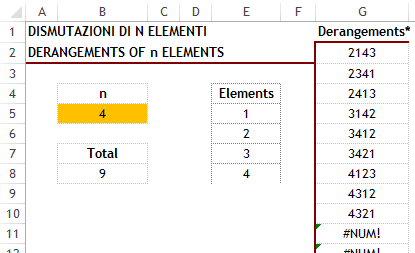Picture 6

I will use the same name n as defined above for the number of elements, and the named formula disp_2 which creates an array for the sequences without repetition, but in this case I have modified it a bit, because now we do not have the k parameter:

`=1+MOD(INT((ROW(OFFSET(\$A\$1,,,n^n))-1)/(n^(COLUMN(OFFSET(\$A\$1,,,,n))-1))),n) `
Here is the array-formula what could be written into a cell and confirm with Ctrl+Shift+Enter, then copy down:

`=SMALL(IF((SUBSTITUTE(MMULT(10^(disp_2),ROW(OFFSET(\$A\$1,,,n))^0),0,"")=REPT(1,n))*(MMULT(--(disp_2=COLUMN(OFFSET(\$A\$1,,,n^n,n))),ROW(OFFSET(\$A\$1,,,n))^0)=0),`
`MMULT(disp_2,10^(n-ROW(OFFSET(\$A\$1,,,n))))),ROW(A1)) `
In this formula I have used two tests in the IF part. The first checks if the sequences have no duplications:

`(SUBSTITUTE(MMULT(10^(disp_2),ROW(OFFSET(\$A\$1,,,n))^0),0,"")=REPT(1,n)) `
(It is explained under the sequences without repetition section.)
The second test determines if any of the elements is in its original position, eg. the natural order:

`(MMULT(--(disp_2=COLUMN(OFFSET(\$A\$1,,,n^n,n))),ROW(OFFSET(\$A\$1,,,n))^0)=0) `
If both tests are passed, so both expressions has TRUE value, I use the row from disp_2 and combine it to decimal number as you have seen in the other formulas:

`MMULT(disp_2,10^(n-ROW(OFFSET(\$A\$1,,,n))))) `

Then comes SMALL and sorts the result.
In the example file you can learn the details of the formula.
The total number of derangements is calculated by n subfactorial ( !n ). The formula calculates this value in Excel could also be interesting:

`=ABS(FACT(n)*SUM((-1^ROW(INDIRECT("1:"&n+1)))/FACT(ROW(INDIRECT("1:"&n+1))-1))) `

## Simplified formulas for 2 element cases

This section is based on the article: https://sites.google.com/site/e90e50/user/calcolo-combinatorio-excel-classe-2

In the previous formulas I used maximum 9 element sets (in particular the numbers from 1 to 9). Now I’d like to focus on the sequences and combinations formed by 2 elements, so the k=2 case. For this case it is possible to develop formulas works with larger sets, where the number of elements are not limited (the only limit is the number of the available rows on the spreadsheet). It also allows us to use the logic that I define “mechanical” and it does not require matrix calculation, though, for fans of the genre, there will be interesting solutions of this kind too.

NB
•In all the examples I use a name range “rng” defined as A2:A16 which contains 15 objects. This range is defined to be static only to speed up the calculation. All formulas will work using a dynamic range (which would allow adding new objects).
•I do not use “n” to represent the number of elements, because in this case n is equal to the number of rows in the named range “rng”, so ROWS(rng) stands for n.
•Please be careful if you need to insert rows - the formulas use references starting from cell A1 - it must not be changed.

### Sequences with repetitions for 2 elements cases

Example file: 2-sequences_with_repetition.xlsx

For 3 (=n) data objects, namely A, B and C the 2-element sequences are: AA, AB, AC, BA, BB, BC, CA, CB, CC.
This is the easiest case, I have built this formula:

`=INDEX(rng,INT((ROW(A1)-1)/ROWS(rng))+1)&";"&INDEX(rng,MOD(ROW(A1)-1,ROWS(rng))+1) `

If you do not want to concatenate the elements, it could be used in two columns:

`=INDEX(rng,INT((ROW(A1)-1)/ROWS(rng))+1) `
`=INDEX(rng,MOD(ROW(A1)-1,ROWS(rng))+1) `

The logic is very simple behind the two formulas and it is obvious when you think about how you write the combinations manually. I use INDEX to choose the first letter from rng as many times as the total number of elements, then I choose the next letter. INT is the formula to create the necessary index numbers. Similarly I choose A, B then C to pair it with letter A, then I choose A, B and C again to pair with letter B - for this case MOD will create the indexes.
The formula producing array result (n * n matrix) is very-very simple:

`=rng & ";" & TRANSPOSE(rng) `

Required to be confirmed with Ctrl + Shift + Enter after selecting a square-shaped range large enough to contain all the results.

### Sequences without repetition for 2 elements cases

Example file: 2-sequences_without_repetition.xlsx

For the same data A, B and C the sequences without repetition are: AB, AC, BA, BC, CA, CB.
The formulas are very similar to the previous one but we must exclude the sequences which contains the same element:
`=INDEX(rng,INT((ROW(A1)-1)/(ROWS(rng)-1))+1)&";"&INDEX(rng,MOD(ROW(A1)-1,ROWS(rng)-1)`
`+2-(MOD(ROW(A1)-1,ROWS(rng)-1)<INT((ROW(A1)-1)/ (ROWS(rng)-1)))) `

The first INDEX is simple, in this case we need the first letter n-1 times. The second index is a bit more complex, it is designed to leave out those numbers which go for the the same letter, causing duplication.
The array formula should also be changed to exclude the equal elements:
`=IF(rng<>TRANSPOSE(rng),rng&TRANSPOSE(rng),NA()) `

It will result an array similar to the previous one but replaces the equal elements with #NA! error value. Needs to be Ctrl+Shift+Entered to a square-shaped range.

### Combinations for 2 elements cases

Example file: 2-combintions_without_repetition.xlsx

For letters A, B and C the 2-element combinations are: AB, AC, BC (in ascending order).
For this case I propose two pair of formulas using help columns and two different array formulas.
As you have seen in the previous solutions, I use INDEX to choose the elements from the list and I concatenate the result of the two INDEX formulas together.
For creating combinations the logic is a bit different. I have used a help column to store a combination of the index numbers. It is necessary because first I generate all the indexes then I replace with empty string those which results duplicated values or combinations not in ascending order. When I use the indexes to generate the combinations from rng I exclude the empty cells with the help of SMALL function. Then I retrieve the index from the combinations separating the two index numbers.
According to the columns in my file, let’s see the formulas.

The first formula I use in column C in the example file:
`=IF(MOD(ROW(A1)-1,ROWS(rng)-1)<INT((ROW(A1)-1)/(ROWS(rng)-1)),"",`
`10^4*(INT((ROW(A1)-1)/ (ROWS(rng)-1))+1)+(MOD(ROW(A1)-1,ROWS(rng)-1)+2)) `

Here I combine the index numbers together by simply adding them as powers of 10 (first number multiplied by 10^4 as 3 digits are enough for separation.)

The formula in column D separates the indexes (dividing by 10^4) and use on rng:
`=INDEX(rng,INT(SMALL(\$C:\$C,ROW(A1)))/10^4)&";"&INDEX(rng,--RIGHT(SMALL(\$C:\$C,ROW(A1)),4)) `

For the other two formulas I use a funny trick to combine then separate the indexes, namely the TIME, MINUTE and SECOND functions.
The first formula combines the index numbers as minutes and seconds - that is why this solution could work only for maximum 60 elements. In the file you find it in column E:
`=IF(MOD(ROW(A1)-1,ROWS(rng)-1)<INT((ROW(A1)-1)/(ROWS(rng)-1)),"",`
`TIME(0,INT((ROW(A1)-1)/ (ROWS(rng)-1))+1,(MOD(ROW(A1)-1,ROWS(rng)-1)+2))) `

And to separate the minutes from seconds and use in INDEX - in the file it is in column F:
`=INDEX(rng,MINUTE(SMALL(\$E:\$E,ROW(A1))))&";"&INDEX(rng,SECOND(SMALL(\$E:\$E,ROW(A1)))) `

The formula which results an array is much more simpler:
`=IF(rng>TRANSPOSE(rng),TRANSPOSE(rng)&";"&rng,NA()) `

The result is a lower triangular matrix, because I exclude the repetitions (diagonal elements) and the permutations too. Needs to be Ctrl+Shift+Entered to a square-shaped range.
And the last formula:
`=INDEX(rng&";"&TRANSPOSE(rng),SMALL(IF(ROW(rng)<TRANSPOSE(ROW(rng)),`
`ROW(rng)-MIN(ROW(rng))+1),ROW(A1)),--RIGHT(SMALL(IF(ROW(rng)`
`<TRANSPOSE(ROW(rng)),--((ROW(rng)-MIN(ROW(rng))+1)&`
`TEXT(TRANSPOSE(ROW(rng)-MIN(ROW(rng))+1),"0000"))),ROW(A1)),4)) `

is also an array-formula, confirm with Ctrl+Shift+Enter into a cell and copy down. Using INDEX it reads the appropriate combinations from the matrix contains all the combinations.

## Examples

### Visualize elements and positions with dynamic chart

Example file: Visualize_english.xlsm

When we write up the sequences, combinations, derangements we focus on the elements of the base set. In the charts you can see the problem from a different perspective: I try to show the position of a particular element within the result sequences. I found it very interesting so I build up a dynamic chart using the mouse rollover technique by Jordan Goldmeier* to visualize the patterns of the elements and positions too.
In the file you can select which kind of method (sequence, combination, etc) you would like to see. The (1) methods created to visualize the positions, the (2) methods visualize the elements, so the “usual” view.
I do not want to go into details, I would like to let you discover the patterns and rules. I only give one example here to make it clear what is happening in the file. Please note, this visualization technique may not be useful and evident for all the cases - in the with repetition methods it may be confusing.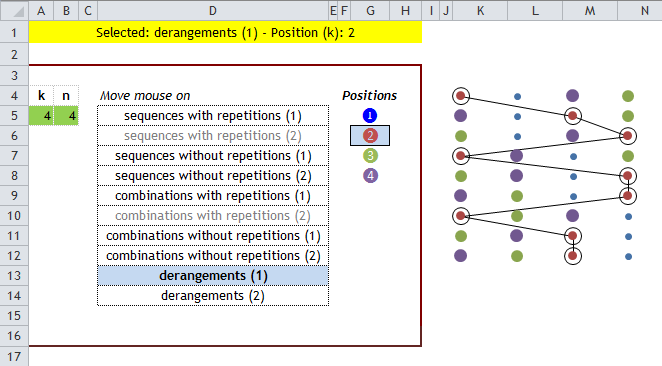Picture 7

On Picture 7 you can see Derangements of 4 elements from “position” point of view. Each column represent one element while the dots represent the positions. Position 2 is selected - the line clearly shows that the second element is never in the second position, according to the rules of derangements.

* Link to Jordan's mouse rollover post on his site.

### Visualize elements with conditional formatting

Example file: Visualize_english_cond_format.xlsm

Reading this Wikipedia article I was inspired to create another visualization using conditional formatting.
This kind of structure makes easier to understand the "with repetitions" cases as you can see on the below picture.
You can see which elements used in the result rows and can count and highlight the elements of the result set.

Picture 8

Also i
n this case we use
Jordan's mouse rollover technique.

### Work with non-numeric data

Example file: Combinations_non_numeric_example.xlsx

The above formulas designed to work with numbers, namely numbers from 1 to 9. We can easily use the result to combine non-numeric data for example with the help of MID and INDEX functions. In the file you can find a general solution which could be used for each of the cases described here. You only have to replace the formula in column F with the one generates the decimal numbers according to your needs.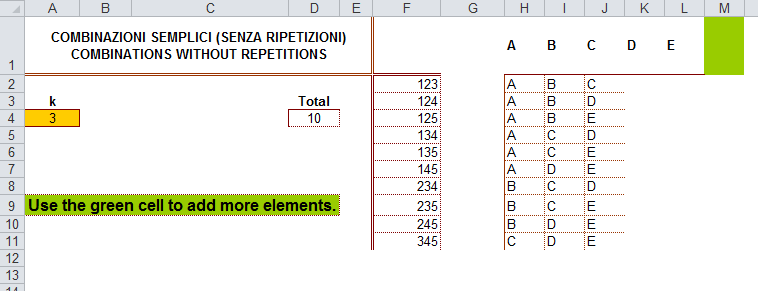Picture 9

It is only one possibility - you can build similar solutions using the separated number-generating formulas too.

### Combine elements of different sets

This section based on a formula challenge and its solutions: https://sites.google.com/site/e90e50fx/home/combinations-excel-formula-challenge
Example file: Combine_sets.xlsx

The above formula were developed to combine the elements of one set. I have developed formulas for combine elements of more sets, when each of the sets could contain different number of elements. For example imagine that you have 3 different countries, 2 different categories and 3 years and you have to write all the possible combinations of country-category-year. In total it will be 3*2*3=18 combinations.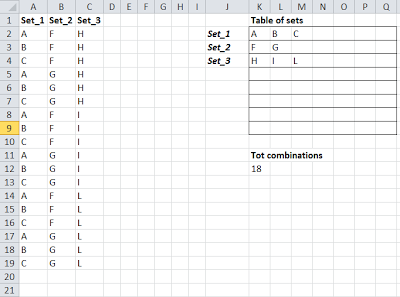Picture 10

In the formulas I use a name tbl_1 for the range containing the sets - in the above screenshot it is K2:Q2 - I use an oversized range to make possible adding new sets and new elements.

First here is a formula to give the number of all possible combinations:
`=PRODUCT(--TEXT(MMULT(1-ISBLANK(tbl_1),TRANSPOSE(COLUMN(tbl_1))^0),"0;;1") `

The problem is seemingly easy, only need to multiply the number of the elements of each set. But using the oversized named range “tbl_1” we face the problem that there are empty rows where the number of elements is 0, so the total product will be 0. To cut this knot I use the TEXT formula to show the 0 values as 1 (This is what the string parameter “0;;1” does - positive numbers appear as integer, negatives appear as empty - in our case no negative value is possible, and the 3rd element of the string is 1 stands for the 0 values.)
I will need the number of combinations to build a formula for the combinations as you can see on the picture in column A, B, C, so I use a named formula tot_comb for the formula above.
Here is the formula for the combinations:

`=IF(ROW(A1)>tot_comb,NA(),INDEX(tbl_1,COLUMN(A1),MOD(INT((ROW(A1)-1)/`
``` PRODUCT(SUBTOTAL(3,OFFSET(tbl_1,ROW(OFFSET(\$A\$1,,,COLUMN(A1),))-2,,1)))),```
``` COUNTA(INDEX(tbl_1,COLUMN(A1),0)))+1)) ```

Confirm with Ctrl+Shift+Enter and copy right and down

## VBA solutions: Anagrams and Combinations

Many times I have had to deal with questions of putting together combinations and permutations.
I have collected some VBA-functions written by me, using regular expressions and dictionary objects to develop anagrams and combinations with repetition.
I publish these not because they would be better than others could be found on the web but in particular because they are special. They have the merit of showing less known mechanisms and techniques related to those objects - that is why I think you will find them interesting.
In the function which creates anagrams of a word, I use the replace method of the RegExp sequences. This technique is not well-documented so I'm sure some of you will find it exciting.
In functions creating combinations I use a for-each loop on a dictionary object that is fed by the cycle itself, a kind of recursion, like the second function but using regular expressions.
The code is commented to explain the way of working.

(It uses “Microsoft Scripting Runtime” in Tools / References...)
If you were able to read until here - you really deserve a special gift ;-)
Belga-Matek

Ĉ
r,
Mar 27, 2013, 4:31 PM
Ĉ
r,
Mar 27, 2013, 4:31 PM
Ĉ
r,
Mar 27, 2013, 4:31 PM
Ĉ
r,
Mar 27, 2013, 4:31 PM
Ĉ
r,
Mar 27, 2013, 4:31 PM
Ĉ
r,
Mar 27, 2013, 4:31 PM
Ĉ
r,
Mar 27, 2013, 4:31 PM
Ĉ
r,
Mar 27, 2013, 4:31 PM
Ċ
r,
Apr 2, 2013, 1:48 AM
Ĉ
r,
Mar 27, 2013, 4:31 PM
Ĉ
r,
Mar 27, 2013, 4:31 PM
Ĉ
r,
Mar 27, 2013, 4:31 PM
Ĉ
r,
Mar 27, 2013, 4:31 PM
Ĉ
r,
Mar 27, 2013, 4:31 PM
Ĉ
r,
Mar 27, 2013, 4:31 PM
Ĉ
r,
Mar 27, 2013, 4:31 PM
Ĉ
r,
Mar 27, 2013, 4:31 PM
Ĉ
r,
Mar 29, 2013, 8:43 AM